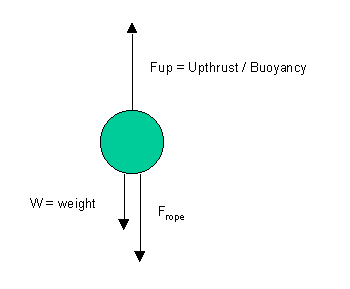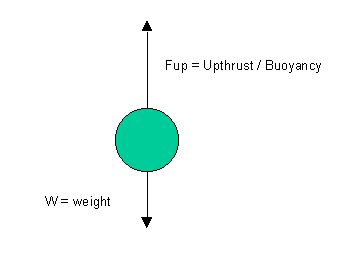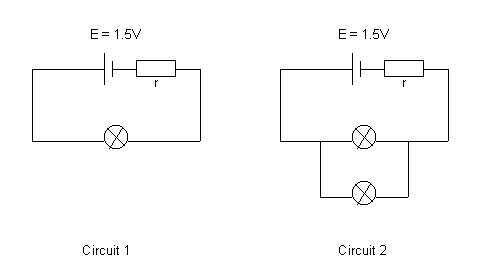# Solutions to SQA examination

### Paper I Solutions

```1. C		11. E		21. C
2. C		12. D		22. E
3. B		13. A		23. A
4. D		14. D		24. B
5. B		15. D		25. E
6. B		16. C		26. A
7. E		17. A		27. A
8. C		18. E		28. B
9. C		19. E		29. B
10.C		20. C		30. E

31.	Step one : calculate the time the arrow takes to reach the target
using the fact that the horizontal velocity is constant.

t = shor/vhor
t = 30/100
t = 0.3s

Step two :  calculate the vertical displacement of the ball in this time.

uver= 0m/s
t = 0.3s
a = -9.8m/s/s
sver = ?

sver = ut + 1/2(at2)
sver = 0 + 1/2(-9.8x0.32)
sver = -0.441m

Step three : calculate the radius of the target.

r = 1.5-0.9
r = 0.6m

As the arrow falls less than the radius of the target it hits
the target.

32.a.i.a.ii.	Frope = Tension(T)

As the rope is stationary the forces acting on the buoy are balanced.

Fup = w + T
Fup = 50 + 1200
Fup = 1250N

b.The buoyancy force is not dependent on depth. Therefore, the buoyancy
force is as calculated in part a.ii.(1250N).

33.In circuit 1 the total resistance in the circuit is equal
to the sum of the internal resistor(r) and the resistance
of the bulb (Rbulb).
Rtotal = r +	Rbulb

In circuit 2 the total resistance in the circuit is equal
to the sum of the internal resistor(r) and the resistance
of the two bulbs in parallel.
Rtotal = r +	Rbulb/2

The total resistance in circuit 2 is less than that in circuit 1. Thus
the current(I) flowing through the internal resistor in circuit 2 is greater.
The "lost"  voltage (Vlost=Ir) across the internal resistor is
therefore greater in circuit 2. This means the voltage across the lamps
(Vlamp = E -Ir) is less in circuit 2 reducing the brightness of the
lamps.

34.a.	Vrms = Vpk/21/2
Vrms = 12/1.414
Vrms = 8.5V

b.	P = Irms2

Irms = Vrms/R
Irms = 8.5/4
Irms = 2.12A

P = 2.122x4
P = 18W

35.	dsinq = nl

d = 1/2.5x105 = 4x10-6m
l = 600x10-9m
n = 1
q = ?

sinq = nl/d
sinq = 1x600x10-9/4x10-6
sinq = 0.15
q = 8.63o

36.a. 	Possible transitions :	E3 -> E2
E3 -> E1
E3 -> E0
E2 -> E1
E2 -> E0
E1 -> E0

b.	A total of 6 lines are in the spectrum.

Low frequency radiation will be produced when the transition
is between energy levels with a small energy gap.

The smallest energy gap is between levels E3 and E2.

c.	Transitions between certain states will be more frequent than others.
The more frequent a transition, the more intense the spectral line will be.

37.	Ek = Ephoton - Ework function

Ephoton = hf = hc/l
Ephoton = (6.63x10-34x3x108)/5.4x10-7
Ephoton = 3.68x10-19J

Ek = 3.7x10-19 - 2.9x10-19
Ek = 0.8x10-19

END OF QUESTION PAPER